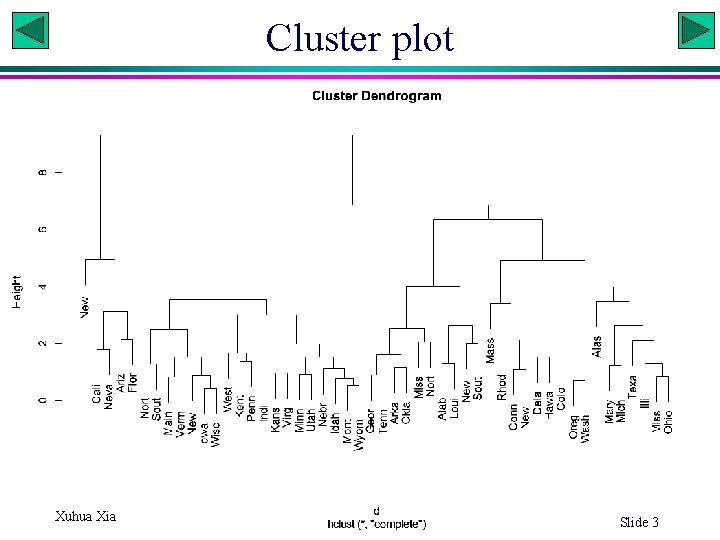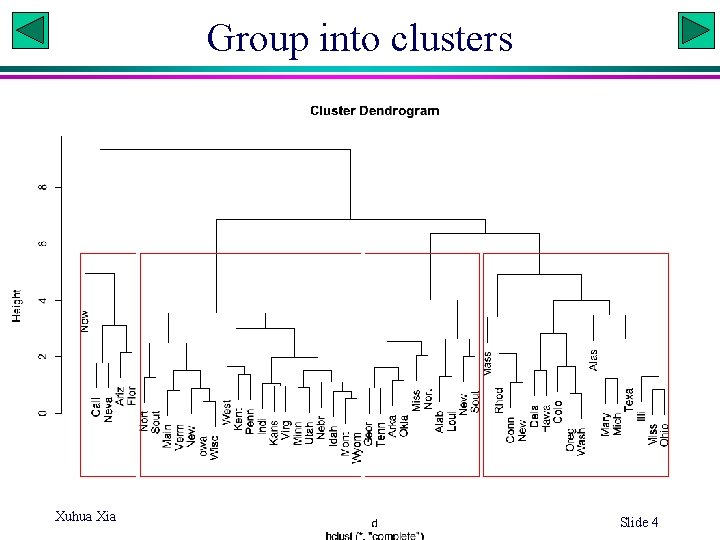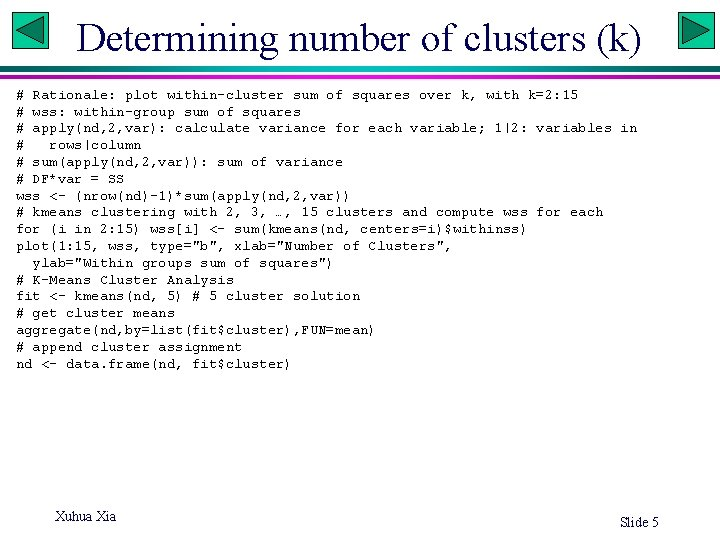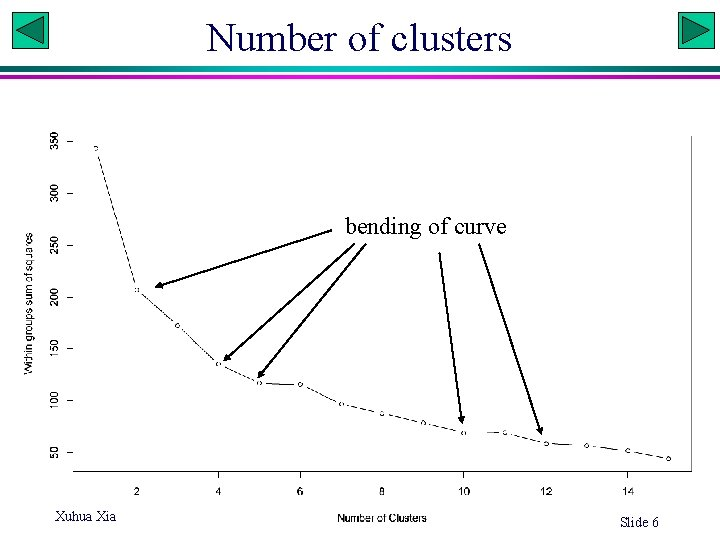# Multivariate statistics PCA principal component analysis Correspondence analysis

• Slides: 6Multivariate statistics • • • PCA: principal component analysis Correspondence analysis Canonical correlation Discriminant function analysis Cluster analysis MANOVA Xuhua Xia Slide 1Cluster analysis. PCA md<read. fwf("http: //dambe. bio. uottawa. ca/teach/bio 8102_download/Crime. txt ", c(14, 6, 5, 6, 6, 7, 7, 6), header=T) nd<-scale(md[, 2: 8]) d<-dist(nd, method="euclidean") # other distances: , "maximum|manhattan|Canberra|binary|minkowski" hc<-hclust(d) s. Label<-substr(md\$STATE, 1, 4) plot(hc, labels=s. Label) rect. hclust(hc, k=4, border="red") Slide 2Cluster plot Xuhua Xia Slide 3Group into clusters Xuhua Xia Slide 4Determining number of clusters (k) # Rationale: plot within-cluster sum of squares over k, with k=2: 15 # wss: within-group sum of squares # apply(nd, 2, var): calculate variance for each variable; 1|2: variables in # rows|column # sum(apply(nd, 2, var)): sum of variance # DF*var = SS wss <- (nrow(nd)-1)*sum(apply(nd, 2, var)) # kmeans clustering with 2, 3, …, 15 clusters and compute wss for each for (i in 2: 15) wss[i] <- sum(kmeans(nd, centers=i)\$withinss) plot(1: 15, wss, type="b", xlab="Number of Clusters", ylab="Within groups sum of squares") # K-Means Cluster Analysis fit <- kmeans(nd, 5) # 5 cluster solution # get cluster means aggregate(nd, by=list(fit\$cluster), FUN=mean) # append cluster assignment nd <- data. frame(nd, fit\$cluster) Xuhua Xia Slide 5Number of clusters bending of curve Xuhua Xia Slide 6